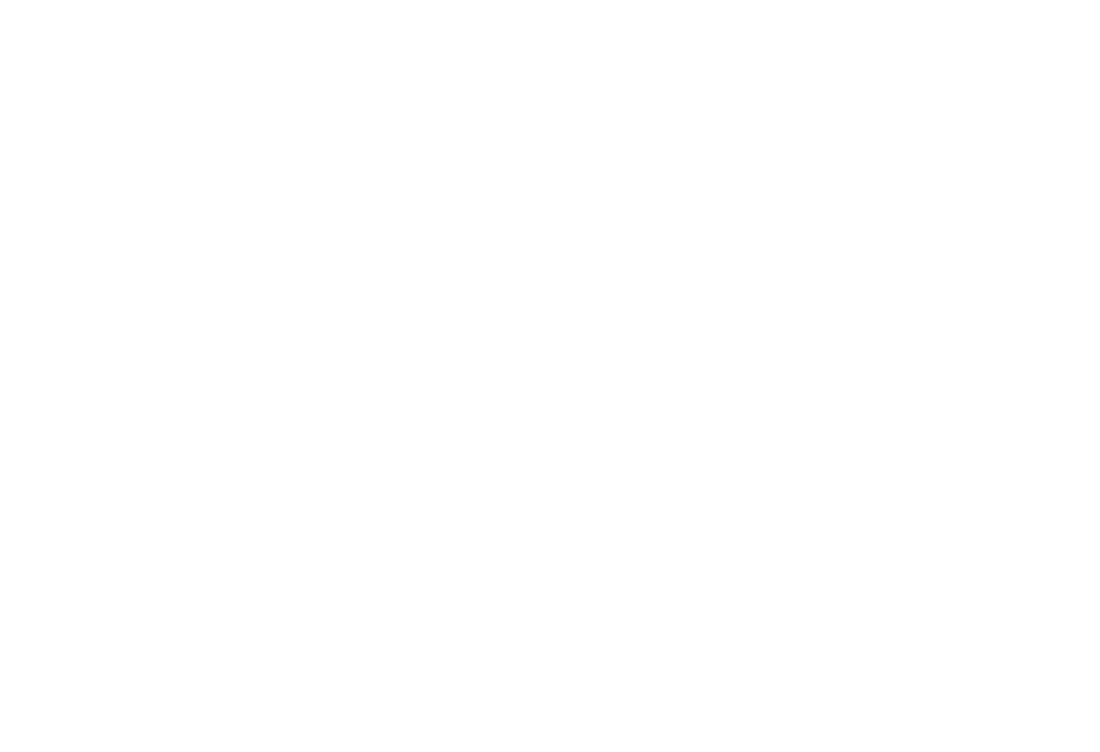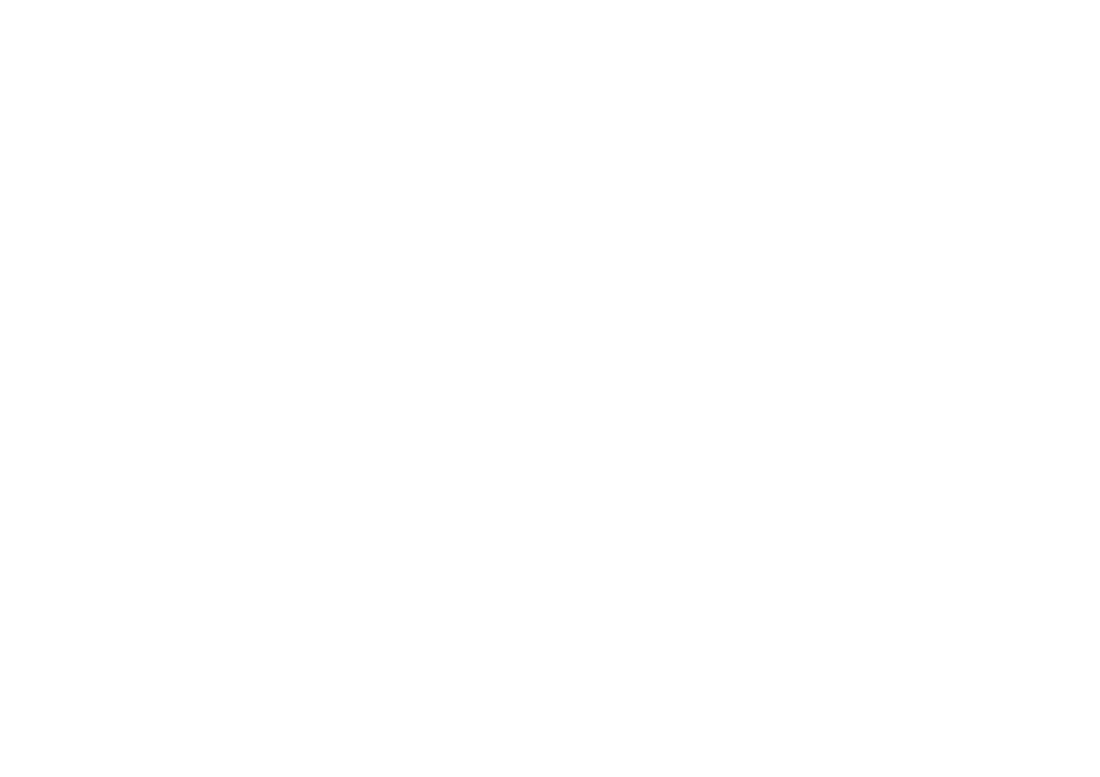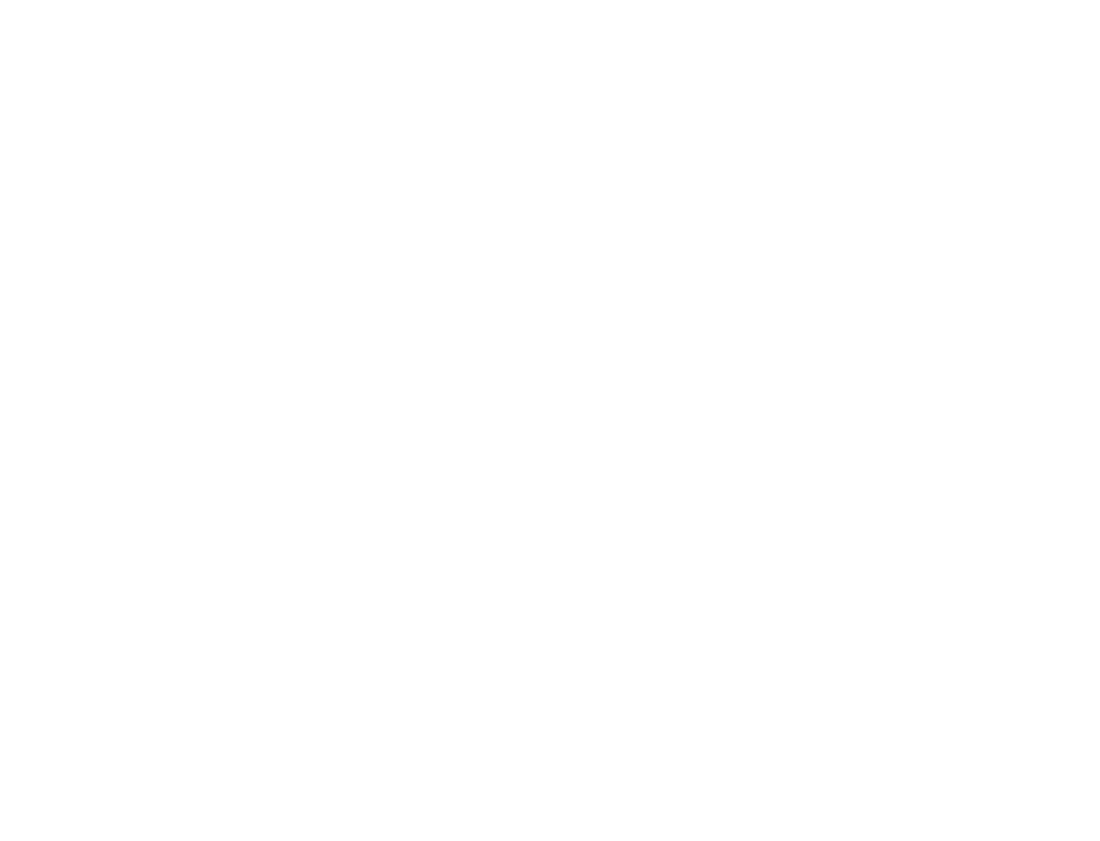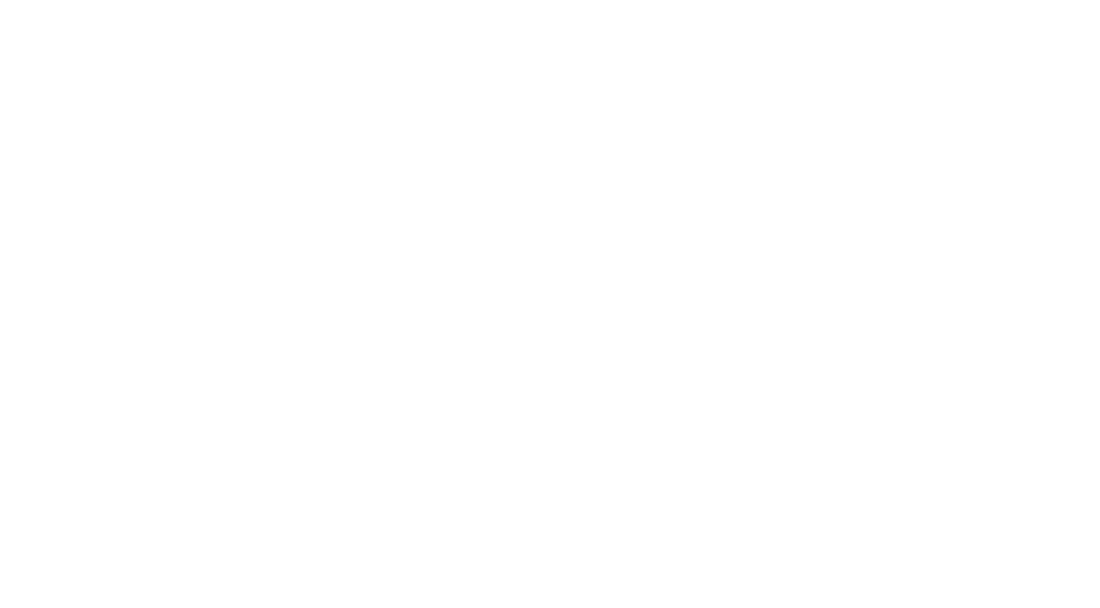# 3.1.2 Wave Effects

### Reflection

• When waves hit an object, such as a barrier, they can be reflected:When waves reflect off a barrier, the angle of reflection, r, is equal to the angle of incidence, i

• When waves are reflection:
`angle of incidence = angle of reflection`
`i = r`

### Refraction

• When waves enter a different medium, their speed can change
• This effect is called refraction, and it can have two other effects:
• The wavelength of the waves can increase or decrease
• The waves can change directionWhen water waves travel from deep areas to shallow areas they slow down

• If the waves slow down the waves will bunch together, causing the wavelength to decrease. The waves will also start to travel closer to the normal
• If the waves speed up then they will spread out, causing the wavelength to increase. The waves will also turn slightly away from the normal

### Diffraction

• When waves pass through a narrow gap, the waves spread out
• This effect is called diffractionDiffraction: when a wave passes through a narrow gap, it spreads out

Extended Only

### Factors Affecting Diffraction

• Diffraction, as shown above, only generally happens when the gap is smaller than the wavelength of the wave
• As the gap gets bigger, the effect gradually gets less pronounced until, in the case that the gap is very much larger than the wavelength, the waves no longer spread out at allThe size of the gap (compared to the wavelength) affects how much the waves spread out

• Diffraction can also occur when waves pass an edgeWhen a wave goes past the edge of a barrier, the waves can curve around the edge

### Demonstrating Wave Effects

#### Exam Tip

When drawing waves being reflected take care to:

• Make sure that the angle of incidence is equal to the angle of reflection
• Keep the wavelength of the waves the same

Similarly, when waves are diffracted the wavelength remains constant.

Refraction is the one wave effect where the wavelength changes.

Remember:
Refraction is the name given to the change in the speed of a wave when it passes from one medium to another. The change in direction is a consequence of this.### Author: Jenna

Jenna studied at Cardiff University before training to become a science teacher at the University of Bath specialising in Biology (although she loves teaching all three sciences at GCSE level!). Teaching is her passion, and with 10 years experience teaching across a wide range of specifications – from GCSE and A Level Biology in the UK to IGCSE and IB Biology internationally – she knows what is required to pass those Biology exams.
CloseClose